In the previous lesson: “How To Convert A Glasses Prescription to Contact Lenses“, I covered only situations that did not involve any astigmatism correction. In this lesson, I will only be discussing how to convert a glasses prescription to contact lenses when there is astigmatism to deal with.

Before I get into it I just want to say that some of this may appear to be difficult. Sure, it does take a little longer, but it’s literally nothing more than a little bit of addition, subtraction, as well as looking up numbers on our vertex distance conversion chart.

Here it is again for you to reference throughout this lesson.

Now let’s get right into it. Everything is done one eye at a time so complete steps 1- 5 for the right eye and then again for the left eye.

Here is the glasses prescription we will be working with:# Step 1: Draw 180° and 90° Reference Lines

The power cross is the main tool used in visualizing and organizing the calculations involved in this conversion process.

Here we will draw two reference lines 180° and 90°.Think of this cross as overlaying the lens of the glasses prescription you’re working with, like this:# Step 2: Add The Sphere Power Line

Look at the glasses prescription that you’re working with and locate the ‘Axis’ number. This number represents a degree value and will be somewhere between 1 and 180. Now draw a line in the direction of the Axis .

In our example prescription, that’s 45. That means that a line at 45° must be drawn on the power cross. This is the Sphere Power Line. It represents the sphere power so write it down next to that line.# Step 3: Add The Sphere+Cylinder Power Line

Next add 90 to the axis number and draw another line in that direction. For the example prescription above that would be 90 + 45 = 135.

This new line is the Sphere+Cylinder Line.

Now, as the name implies, to find the power of this line we must add the sphere and cylinder together.

For the example prescription above that would be -8.50 + (-1.00) = -9.50.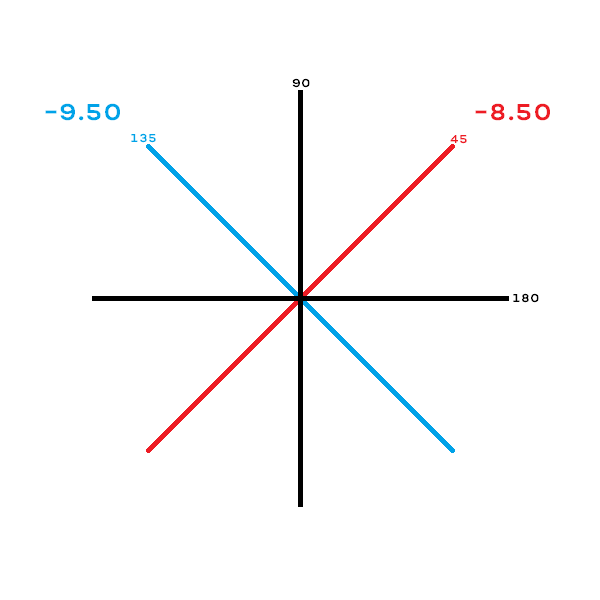This the completed power cross.

# Step 4: Covert The Powers

This is the step where we actually convert the glasses prescription to contact lenses. Now that we’ve laid out both the sphere and the sphere+cylinder powers, use the vertex distance conversion chart above to convert them and write those down as well.# Step 5: Returning To Prescription Format

At this point, the glasses prescription is converted to contact lenses, but it’s not in the correct Sphere/Cylinder/Axis format. To return to the correct format, follow these 3 rules:

1. The converted sphere power becomes the contact lens sphere power.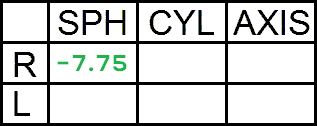2. The converted sphere+cylinder number minus the converted sphere number becomes the contact lens cylinder power.

#### -8.50 – (-7.75) = -0.75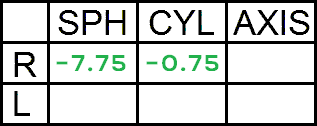3. The original glasses axis becomes the contact lens axis (it doesn’t change)# And that’s it!

You’ve done it! Not bad right?

Let’s work through the left eye together.# Step 1: Draw 180° and 90° Reference Lines# Step 2: Add The Sphere Power Line

This time the axis is 020 so the sphere power line must be drawn at 20°. The sphere power is -7.00 so that will go next to the sphere power line.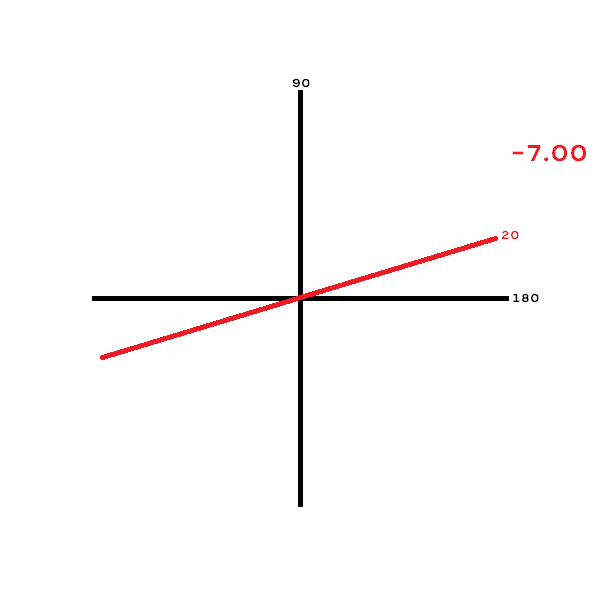# Step 3: Add The Sphere+Cylinder Power Line

Next, add 90 to the axis on the prescription. In this case it’s 90 + 20 = 110, so the sphere+cylinder power line must be drawn at 110°.

The sphere+cylinder power for this prescription is -7.00 + (-1.50) = -8.50, so that will go next to the sphere+cylinder line.# Step 4: Covert The Powers

The vertex distance conversion chart is now used to convert both the sphere power and the sphere+cylinder power. The converted powers are written down to help with the next step.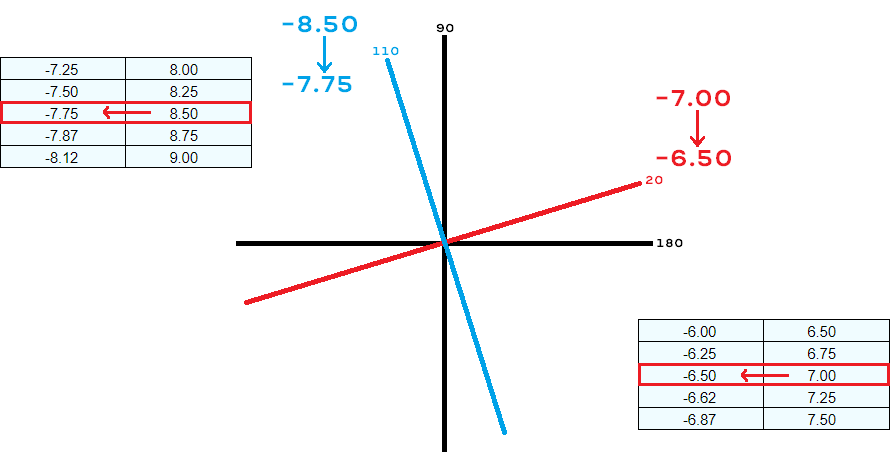# Step 5: Returning To Prescription Format

1. The converted sphere power becomes the contact lens sphere power.2. The converted sphere power minus the converted sphere+cylinder power becomes the contact lens cylinder power.

#### -7.75 – (-6.50) = -1.25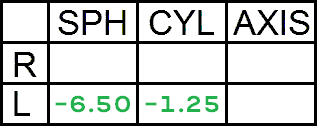3. The original glasses axis becomes the contact lens axis (it doesn’t change).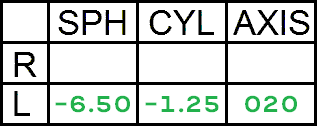And that’s how to convert a glasses prescription to contact lenses!

# Important Shortcuts

Despite the simplicity of converting a glasses prescription with astigmatism to contact lenses using the 5 step process outlined above, it’s not always necessary. There are some shortcuts that can save a lot of time in a real-world practice setting.

### When The Glasses Cylinder is -0.25

If the cylinder on the glasses prescription that is being converted to contact lenses is -0.25, there is no need to go through the 5-step power cross procedure. In fact, most of the time there is no need to do anything at all.

With a cylinder of -0.25, after the conversion process the cylinder will either stay -0.25 or become even less. Since there are absolutely no contact lens company that offer a contact lens for astigmatism with a cylinder correction of -0.25, it is a pointless exercise to convert.

Instead, the most common solution is to ignore it altogether. Simply, the cylinder and axis are removed from the contact lens prescription and only the glasses sphere is converted according to these guidelines.

### When The Glasses Cylinder is -0.50

This is perhaps the trickiest situation. Contact lenses are not available in cylinder power of -0.50, yet, a cylinder power of -0.50 is significant enough that it can’t just be ignored. So what is the solution?

The -0.50 cylinder & axis are still dropped from the contact lens prescription, however, to compensate for it, and additional -0.25 is added to the glasses sphere. That is, you do not have to use the power cross method. Simply:

1. Remove the -0.50 cylinder & axis.
2. Add -0.25 to the glasses sphere.
3. Convert the new glasses sphere using a vertex distance conversion chart.

*Side note, this is called taking the spherical equivalent of a prescription.

Here’s an example:1. Remove the -0.50 cylinder & axis.2. Add -0.25 to the glasses sphere.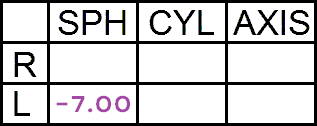3. Convert the new glasses sphere using a vertex distance conversion chart.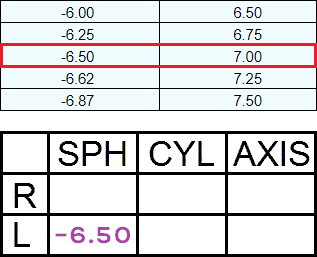And there you have it. You now have all the tools and knowledge necessary to convert any glasses prescription to contact lenses. But are you now ready to start prescribing contact lenses? Not quite yet! Actually, at this point we’re still in the very early stages of the contact lens fitting.

# Next Steps

You know how to convert a glasses prescription to contact lenses, even if it has astigmatism, but what if contact lenses aren’t available in the numbers that you calculated?

Have you noticed that many of the conversions on the vertex distance conversion chart end in weird numbers like 0.12, 0.37, 0.62, & 0.87? Have you noticed that only certain cylinder powers and axis are available for contact lenses?

If the conversion you calculated doesn’t match what is available in contact lenses, there will be additional adjustments needed in order for trials to be ordered for the patient/client. And that’s what we’re going to learn about next!